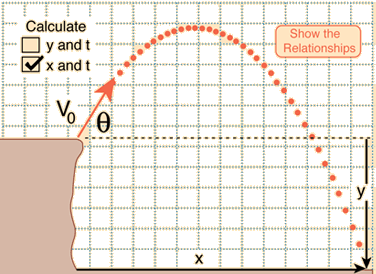# A water balloon is thrown by Sandra out of her dormitory window at an angle of 60o. It lands at the feet of Chris, who is standing a horizontal distance of 6.00 m from the building. If it takes 2.50 s to reach Chris’s feet ?

## how to determine the height h that Sandra threw it from.

Dec 13, 2015

$20.2 \text{m}$

#### Explanation:

The situation is like this:In the convention I use, up is +ve, down is -ve.

$\theta = {60}^{\circ}$

$x = 6 \text{m}$

The horizontal component of the velocity is constant so:

$v \cos 60 = \frac{x}{t}$

$\therefore v = \frac{x}{t \cos 60} = \frac{6}{2.5 \times 0.5} = 4.8 \text{m/s}$

Now we can use:

$s = u t + \frac{1}{2} a {t}^{2}$

Using the sign convention this becomes:

$h = v \sin \theta t - \frac{1}{2} \text{g} {t}^{2}$

$\therefore h = 4.8 \times 0.866 \times 2.5 - 0.5 \times 9.8 \times {2.5}^{2}$

$h = 10.392 - 30.625 = - 20.233 \text{m}$

This means that Sandra threw it from a height of $20.2 \text{m}$